Tamilnadu State Board New Syllabus Samacheer Kalvi 8th Maths Guide Pdf Chapter 1 Numbers Ex 1.2 Textbook Questions and Answers, Notes.

## Tamilnadu Samacheer Kalvi 8th Maths Solutions Chapter 1 Numbers Ex 1.2

Question 1.
Fill in the blanks:

(i) The value of $$\frac{-5}{12}+\frac{7}{15}$$ = ________ .
$$\frac{1}{20}$$

(ii) The value of $$\left(\frac{-3}{6}\right) \times\left(\frac{18}{-9}\right)$$ is = ________ .
1(iii) The value of $$\left(\frac{-15}{23}\right) \div\left(\frac{30}{-46}\right)$$ is ________ .
1

(iv) The rational number ________ does not have a reciprocal.
0

(v) The multiplicative inverse of -1 is ________ .
-1

Question 2.
Say True or False
(i) All rational numbers have an additive inverse.
True(ii) The rational numbers that are equal to their additive inverses are 0 and -1.
False

(iii) The additive inverse of $$\frac{-11}{-17}$$ is $$\frac{11}{17}$$
False

(iv) The rational number which is its own reciprocal is -1.
True

(v) The multiplicative inverse exists for all rational numbers.
False

Question 3.
Find the sum
(i) $$\frac{7}{5}+\frac{3}{5}$$
(ii) $$\frac{7}{5}+\frac{5}{7}$$
(iii) $$\frac{6}{5}+\left(\frac{-14}{15}\right)$$
(iv) $$-4 \frac{2}{3}+7 \frac{5}{12}$$
(i) $$\frac{7}{5}+\frac{3}{5}$$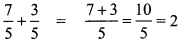(ii) $$\frac{7}{5}+\frac{5}{7}$$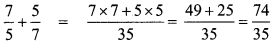(iii) $$\frac{6}{5}+\left(\frac{-14}{15}\right)$$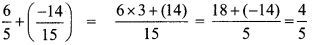(iv) $$-4 \frac{2}{3}+7 \frac{5}{12}$$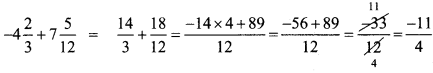Question 4.
Subtract $$\frac{-8}{44}$$ from $$\frac{-17}{11}$$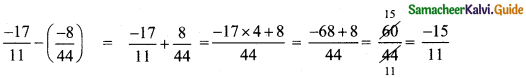Question 5.
Evaluate
(i) $$\frac{9}{132} \times \frac{-11}{3}$$
(ii) $$\frac{-7}{27} \times \frac{24}{-35}$$
(i) $$\frac{9}{132} \times \frac{-11}{3}$$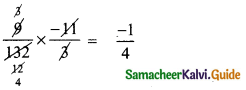(ii) $$\frac{-7}{27} \times \frac{24}{-35}$$Question 6.
Divide
(i) $$\frac{-21}{5}$$ by $$\frac{-7}{-10}$$
(ii) $$\frac{-3}{13}$$ by -3
(iii) -2 by $$\frac{-6}{15}$$
(i) $$\frac{-21}{5}$$ by $$\frac{-7}{-10}$$(ii) $$\frac{-3}{13}$$ by -3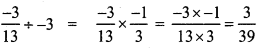(iii) -2 by $$\frac{-6}{15}$$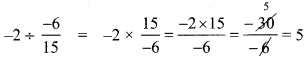Question 7.
Find (a + b) ÷ (a – b) if
(i) a = $$\frac{1}{2}$$, b = $$\frac{2}{3}$$
(ii) a = $$\frac{-3}{5}$$, b = $$\frac{2}{15}$$
(i) a = $$\frac{1}{2}$$, b = $$\frac{2}{3}$$(ii) a = $$\frac{-3}{5}$$, b = $$\frac{2}{15}$$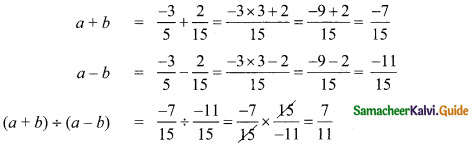Question 8.
Simplify $$\frac{1}{2}+\left(\frac{3}{2}-\frac{2}{5}\right) \div \frac{3}{10} \times 3$$ and show that it is a rational number between 11 and 12.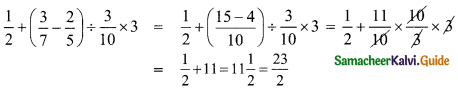Question 9.
Simplify
(i) $$\left[\frac{11}{8} \times\left(\frac{-6}{33}\right)\right]+\left[\frac{1}{3}+\left(\frac{3}{5} \div \frac{9}{20}\right)\right]-\left[\frac{4}{7} \times \frac{-7}{5}\right]$$
(ii) $$\left[\frac{4}{3} \div\left(\frac{8}{-7}\right)\right]-\left[\frac{3}{4} \times \frac{4}{3}\right]+\left[\frac{4}{3} \times\left(\frac{-1}{4}\right)\right]$$
(i) $$\left[\frac{11}{8} \times\left(\frac{-6}{33}\right)\right]+\left[\frac{1}{3}+\left(\frac{3}{5} \div \frac{9}{20}\right)\right]-\left[\frac{4}{7} \times \frac{-7}{5}\right]$$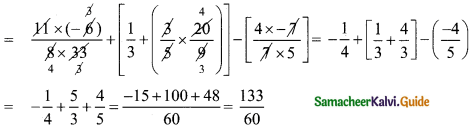(ii) $$\left[\frac{4}{3} \div\left(\frac{8}{-7}\right)\right]-\left[\frac{3}{4} \times \frac{4}{3}\right]+\left[\frac{4}{3} \times\left(\frac{-1}{4}\right)\right]$$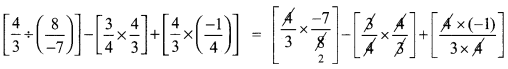Question 10.
A student had multiplied a number by $$\frac{4}{3}$$ instead of dividing it by $$\frac{4}{3}$$ and got 70 more than the correct answer. Find the number.
Let the number = a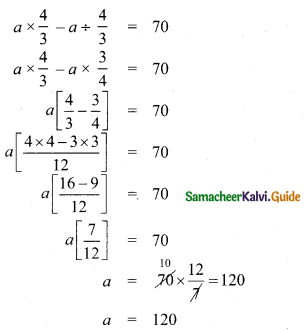Objective Type Questions

Question 11.
The standard form of the sum  is ________ .
(A) 1
(B) $$\frac{-1}{2}$$
(C) $$\frac{1}{12}$$
(D) $$\frac{1}{22}$$
1
Hint:Question 12.
$$\left(\frac{3}{4}-\frac{5}{8}\right)+\frac{1}{2}$$ = _______ .
(A) $$\frac{15}{64}$$
(B) 1
(C) $$\frac{5}{8}$$
(D) $$\frac{1}{16}$$
(C) $$\frac{5}{8}$$
Hint:Question 13.
$$\frac{3}{4}+\left(\frac{5}{8}+\frac{1}{2}\right)$$ =
(A) $$\frac{13}{10}$$
(B) $$\frac{2}{3}$$
(C) $$\frac{3}{2}$$
(D) $$\frac{5}{8}$$
(B) $$\frac{2}{3}$$
Hint:Question 14.
 = _______ .
(A) $$\frac{5}{8}$$
(B) $$\frac{2}{3}$$
(C) $$\frac{15}{32}$$
(D) $$\frac{15}{16}$$
(D) $$\frac{15}{16}$$
Hint:Question 15.
Which of these rational number which have additive inverse?
(A) 7
(B) $$\frac{-5}{7}$$
(C) 0
(D) all of these
Additive inverse of $$\frac{-5}{7}$$ is $$\frac{5}{7}$$